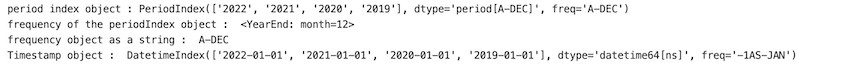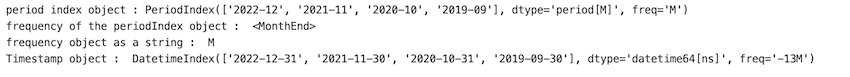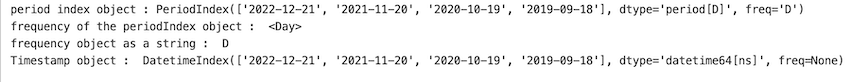# Python Pandas – Convert PeriodIndex object to Timestamp and set the frequency

• Last Updated : 28 Feb, 2022

In this article, we will discuss how to convert period index objects to timestamp and set the frequency in the python programming language.

The pandas PeriodIndex.to_timestamp() method is used to convert a PeriodIndex object to Timestamp and set the frequency. frequency can be set using the ‘freq’ parameter of the method.

### Example 1:

Pandas package is imported. A period index object is created using pd.PeriodIndex() function where we pass in an array of DateTime values and frequency is specified as “year”. The period index object will have YearEnd type frequency. PeriodIndex object is converted to timestamp object by using the pd.to_timestamp() method.

## Python3

 `# import packages ` `import` `pandas as pd ` ` `  `# Create a PeriodIndex object ` `# freq ='Y' represents year. ` `periodIndex ``=` `pd.PeriodIndex([``'2022-12-21 09:30:20'``, ``'2021-11-20 06:45:40'``, ` `                              ``'2020-10-19 03:38:15'``, ``'2019-09-18 01:30:30'``], ` `                             ``freq``=``"Y"``) ` ` `  `print``(``'period index object : '` `+` `str``(periodIndex)) ` `print``(``"frequency of the periodIndex object : "``, periodIndex.freq) ` ` `  `# Display PeriodIndex frequency as string ` `print``(``"frequency object as a string : "``, periodIndex.freqstr) ` ` `  `# Converting PeriodIndex object to timestamp ` `print``(``"Timestamp object : "``, periodIndex.to_timestamp()) `

Output:Output

### Example 2:

In this example, we give the string “M” as the frequency which gives us a period index object of type “MonthEnd”. we also specify the time stamp object to have a frequency of “M”.

## Python3

 `#import packages ` `import` `pandas as pd ` ` `  `# Create a PeriodIndex object ` `# freq ='Y' represents month. ` `periodIndex ``=` `pd.PeriodIndex([``'2022-12-21 09:30:20'``, ``'2021-11-20 06:45:40'``, ` `                              ``'2020-10-19 03:38:15'``, ``'2019-09-18 01:30:30'``],  ` `                             ``freq``=``"M"``) ` ` `  `print``(``'period index object : '` `+` `str``(periodIndex)) ` `print``(``"frequency of the periodIndex object : "``, periodIndex.freq) ` ` `  `# Display PeriodIndex frequency as string ` `print``(``"frequency object as a string : "``, periodIndex.freqstr) ` ` `  `# Converting PeriodIndex object to timestamp ` `print``(``"Timestamp object : "``, periodIndex.to_timestamp(freq``=``'M'``)) `

Output:Output

### Example 3:

In this example, we give the string “D” as the frequency which gives us a period index object of type “Day”. we also specify the time stamp object to have a frequency of “D”. The period Index object values match exactly with the values in the Timestamp object as we specify frequency to be “Day”.

## Python3

 `# import packages ` `import` `pandas as pd ` ` `  `# Create a PeriodIndex object ` `# freq ='Y' represents Day. ` `periodIndex ``=` `pd.PeriodIndex([``'2022-12-21 09:30:20'``, ``'2021-11-20 06:45:40'``, ` `                              ``'2020-10-19 03:38:15'``, ``'2019-09-18 01:30:30'``], ` `                             ``freq``=``"D"``) ` ` `  `print``(``'period index object : '` `+` `str``(periodIndex)) ` `print``(``"frequency of the periodIndex object : "``, periodIndex.freq) ` ` `  `# Display PeriodIndex frequency as string ` `print``(``"frequency object as a string : "``, periodIndex.freqstr) ` ` `  `# Converting PeriodIndex object to timestamp ` `print``(``"Timestamp object : "``, periodIndex.to_timestamp(freq``=``'D'``)) `

Output:Output

My Personal Notes arrow_drop_up
Recommended Articles
Page :